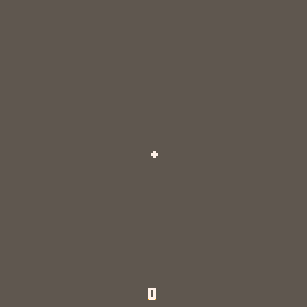Color Dodge is a very small game I made for TweetTweetJam 5 ! The main rule is pretty simple, the final game code must be 560 characters or less. So I decided to challenge my few Pico8 skills to make a game where you have to keep dodging the incoming explosions for as much time as possible !```r,c=rnd,circfill::w::x,y,t,u,b=64,64,0,0,{}::_::cls(5)t+=1u+=1z=btn()x+=z\2%2-z%2y+=z\8%2-z\4%2if t>18then
t=0end
for g in all(b)do
g.t+=1if g.t>32then g.a+=1
if(g.a>g.m)del(b,g)
l={2,8,9,10,9,8,2,1}g.c=l[1+flr((g.a/g.m)*5)]g.s=(1-(g.a/g.m))*g.is g.e*=0.9g.f*=0.9g.x+=g.e g.y+=g.f
if((x-g.x)*(x-g.x)+(y-g.y)*(y-g.y)<g.s*g.s)goto w
else c(g.x,g.y,g.t/9,1)end end for g in all(b)do if(g.c!=nil)c(g.x,g.y,g.s,g.c)end
c(x,y,1,7)
?flr(u/30),62,120,7
flip()goto _```

It was hard for me not having enough space to add some great camera shake, but it was really really fun to make !

 Status Prototype Platforms HTML5 Rating Rated 5.0 out of 5 stars(1 total ratings) Author Robin Six Genre Action Made with PICO-8 Tags PICO-8, tweettweetjam